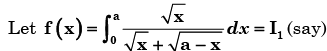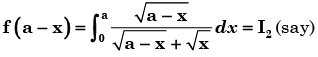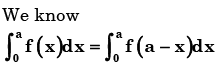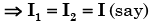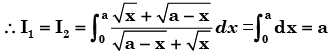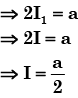Courses

# Calculus, Theorems Of Integral Calculus - MCQ Test 1

## 20 Questions MCQ Test Topicwise Question Bank for GATE Civil Engineering | Calculus, Theorems Of Integral Calculus - MCQ Test 1

Description
This mock test of Calculus, Theorems Of Integral Calculus - MCQ Test 1 for Mechanical Engineering helps you for every Mechanical Engineering entrance exam. This contains 20 Multiple Choice Questions for Mechanical Engineering Calculus, Theorems Of Integral Calculus - MCQ Test 1 (mcq) to study with solutions a complete question bank. The solved questions answers in this Calculus, Theorems Of Integral Calculus - MCQ Test 1 quiz give you a good mix of easy questions and tough questions. Mechanical Engineering students definitely take this Calculus, Theorems Of Integral Calculus - MCQ Test 1 exercise for a better result in the exam. You can find other Calculus, Theorems Of Integral Calculus - MCQ Test 1 extra questions, long questions & short questions for Mechanical Engineering on EduRev as well by searching above.
QUESTION: 1

### As x is increased from – ∞ to ∞ , the function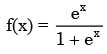Solution: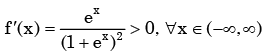QUESTION: 2

###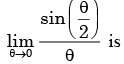Solution: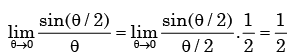QUESTION: 3

###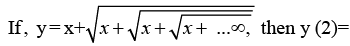Solution:

Given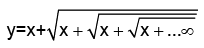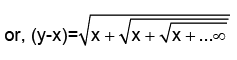Square both side, we get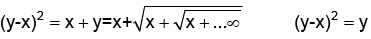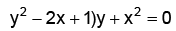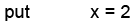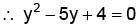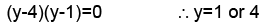QUESTION: 4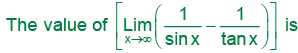Solution: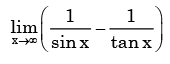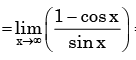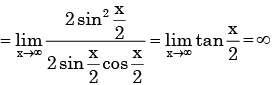QUESTION: 5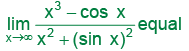Solution:

cos x and sin x are finite whatever x may be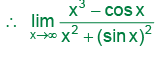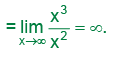QUESTION: 6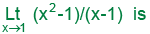Solution: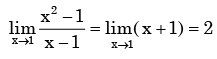QUESTION: 7

Which of the following functions is not differentiable in the domain [-1,1]?

Solution: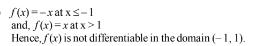QUESTION: 8

If f(x) =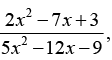then limx-►3 f(x) will be

Solution: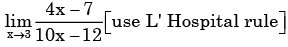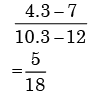QUESTION: 9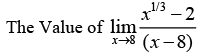Solution: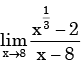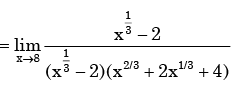= 1/12

QUESTION: 10

What should be the value of λ such that the function defined below is continuous at x = π/2?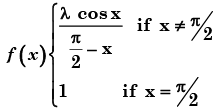Solution:

By the given condition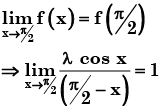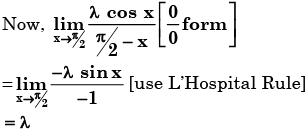From (1), λ = 1

QUESTION: 11

The value of the function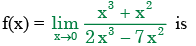Solution: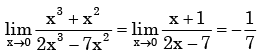QUESTION: 12

Consider the function f(x) = |x|3, where x is real. Then the function f(x) at x = 0 is

Solution: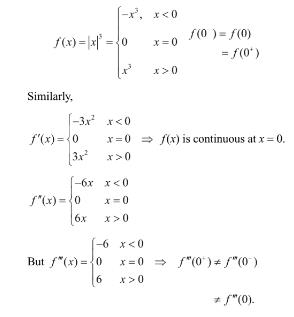QUESTION: 13

The expression e–ln x for x > 0 is equal to

Solution: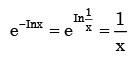QUESTION: 14

Consider the following two statements about the function f(x) = |x|

P: f(x) is continuous for all real values of x

Q: f(x) is differentiable for all real values of x

Which of the f oll owi ng is TRU E?

Solution: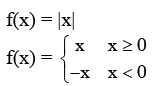The graph of f(x) is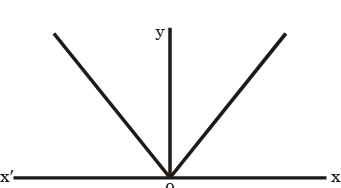f(x) is continuous for all real values of x   Lim |x| = Lim |x| = 0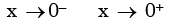as can be seen from graph of |x|.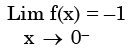and  Lim f(x) = +1 as can be seen from graph of |x|

x → 0+

Left deriva tive ≠ Rig ht derivative

So |x| is continuous but not differentiable at x = 0.

QUESTION: 15

What is the value of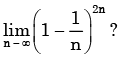Solution: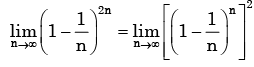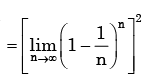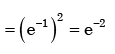QUESTION: 16

The integral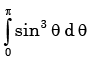is given by

Solution: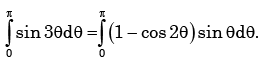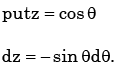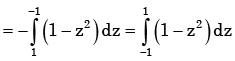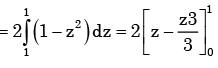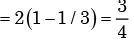QUESTION: 17

Which one of the following function is strictly bounded?

Solution:

For a strictly bounded function f(x), limit should be finite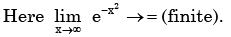QUESTION: 18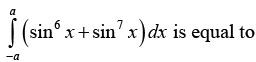Solution: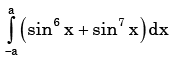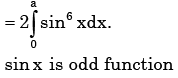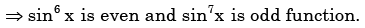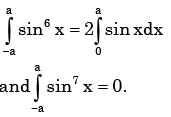QUESTION: 19

Which of the following integrals is unbounded?

Solution: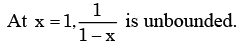QUESTION: 20

What is the value of the definite integral,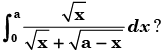Solution: